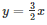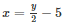Courses

# RD Sharma Solutions Ex-13.1, Linear Equation In Two Variables, Class 9, Maths Class 9 Notes | EduRev

## RD Sharma Solutions for Class 9 Mathematics

Created by: Abhishek Kapoor

## Class 9 : RD Sharma Solutions Ex-13.1, Linear Equation In Two Variables, Class 9, Maths Class 9 Notes | EduRev

The document RD Sharma Solutions Ex-13.1, Linear Equation In Two Variables, Class 9, Maths Class 9 Notes | EduRev is a part of the Class 9 Course RD Sharma Solutions for Class 9 Mathematics.
All you need of Class 9 at this link: Class 9

Q 1: Express the following linear equations in the form ax + by + c = 0 and indicate the values of a, b and c in each case:

(i) -2x + 3y = 12
(ii) x – y/2 – 5 = 0
(iii) 2x + 3y = 9.35
(iv) 3x = -7y
(v) 2x + 3 = 0
(vi) y – 5 = 0
(vii) 4 = 3x
(viii) y = x/2 ;

A 1 :

(i) We are given

– 2x + 3y = 12

– 2x  + 3y – 12 = 0

Comparing the given equation with ax+ by+ c = O

We get, a = – 2; b = 3; c = -12

(ii) We are given

x – y/2 – 5= 0

Comparing the given equation with ax + by + c = 0 ,

We get, a = 1; b = -1/2, c = -5

(iii) We are given

2x + 3y = 9.35

2x + 3y – 9.35 =0

Comparing the given equation with ax + by + c = 0

We get, a = 2 ; b = 3 ; c = -9.35

(iv) We are given

3x = -7y

3x + 7y = 0

Comparing the given equation with ax+ by + c = 0,

We get, a = 3 ; b = 7 ; c = 0

(v) We are given

2x + 3 = 0

Comparing the given equation with ax + by + c = 0,

We get, a = 2 ; b = 0 ; c = 3

(vi) We are given

Y – 5 = 0

Comparing the given equation with ax + by+ c = 0,

We get, a = 0; b = 1; c = -5

(vii) We are given

4 = 3x

3x-4 = 0

Comparing the given equation with ax + by + c = 0,

We get, a = 3; b = 0; c = -4

(viii) We are given

Y = x/2

Taking L.C.M ⇒ x — 2y = 0

Comparing the given equation with ax + by + c = 0 ,

We get, a = 1; b = -2; c = 0

Q 2: Write each of the following as an equation in two variables:

(i) 2x = -3
(ii) y=3
(iii) 5x = 7/ 2
(iv) y = 3/2x

A 2:

(i) We are given,

2x = -3

Now, in two variable forms the given equation will be

2x + 0y + 3 = 0

(ii) We are given,

y = 3

Now, in two variable forms the given equation will be

0 x + y – 3 = 0

(iii) We are given,

5x = -7/2

Now, in two variable forms the given equation will be

5x + Oy +7/2 = 0

10x + Oy – 7  = 0

(iv) We are given,(Taking L.C.M on both sides)

Now, in two variable forms the given equation will be

3x – 2y + 0 = 0

Q 3 :  The cost of ball pen is Rs 5 less than half of the cost of fountain pen. Write this statement as a linear equation in two variables.

A 3:

Let the cost of fountain pen be y and cost of ball pen be x.

According to the given equation, we have⇒ 2x = y – 10

⇒ 2x –y + 10 = 0

Here y is the cost of one fountain pen and x is that of one ball pen.

91 docs

,

,

,

,

,

,

,

,

,

,

,

,

,

,

,

,

,

,

,

,

,

,

,

,

,

,

,

,

,

,

;